# Data Formatting Guide: Ungrouping and Checking Imbalance

• Updated

## Summary

This guide is to help you understand the best ways to troubleshoot an imbalance in the MindBridge results when your GL file is formatted and is able to be easily ingested into MindBridge.

This is a more advanced and comprehensive walkthrough. So if you haven't already, we recommend trying the solutions found in Amounts or Debits and Credits do not balance when importing GL into MindBridge.

## Resolution

Consider the following excerpt from a GL file:First, we will briefly review how to format this file. If you prefer, you can skip over this part and go directly to Troubleshooting the imbalance.

## The problems:

1. This file is grouped by account: only one row contains account information (rows 6, 10, 169), followed by all transactions for that row (rows 12-167, 171 onward) (violates row requirement 1).
2. The account number is usually in column B (rows 10 and 169) but sometimes is in column A (row 6) (violates column requirement 1) (However, account description is always in column E for account rows)
3. There are unneeded total/subtotal rows (9, 168) and blank rows under the account number rows (violates overall requirement 2)

### The solution (formatting needed):

1. Ungroup by account (drag down account information to each transaction row)
2. Exclude extraneous total/subtotal/blank rows (rows 7-9, 11, 168-170) and extraneous rows (rows 1-4) above the header row (row 5)

We add an "Account ID" column at the end of the file to extract the account number from the account rows and drag it down: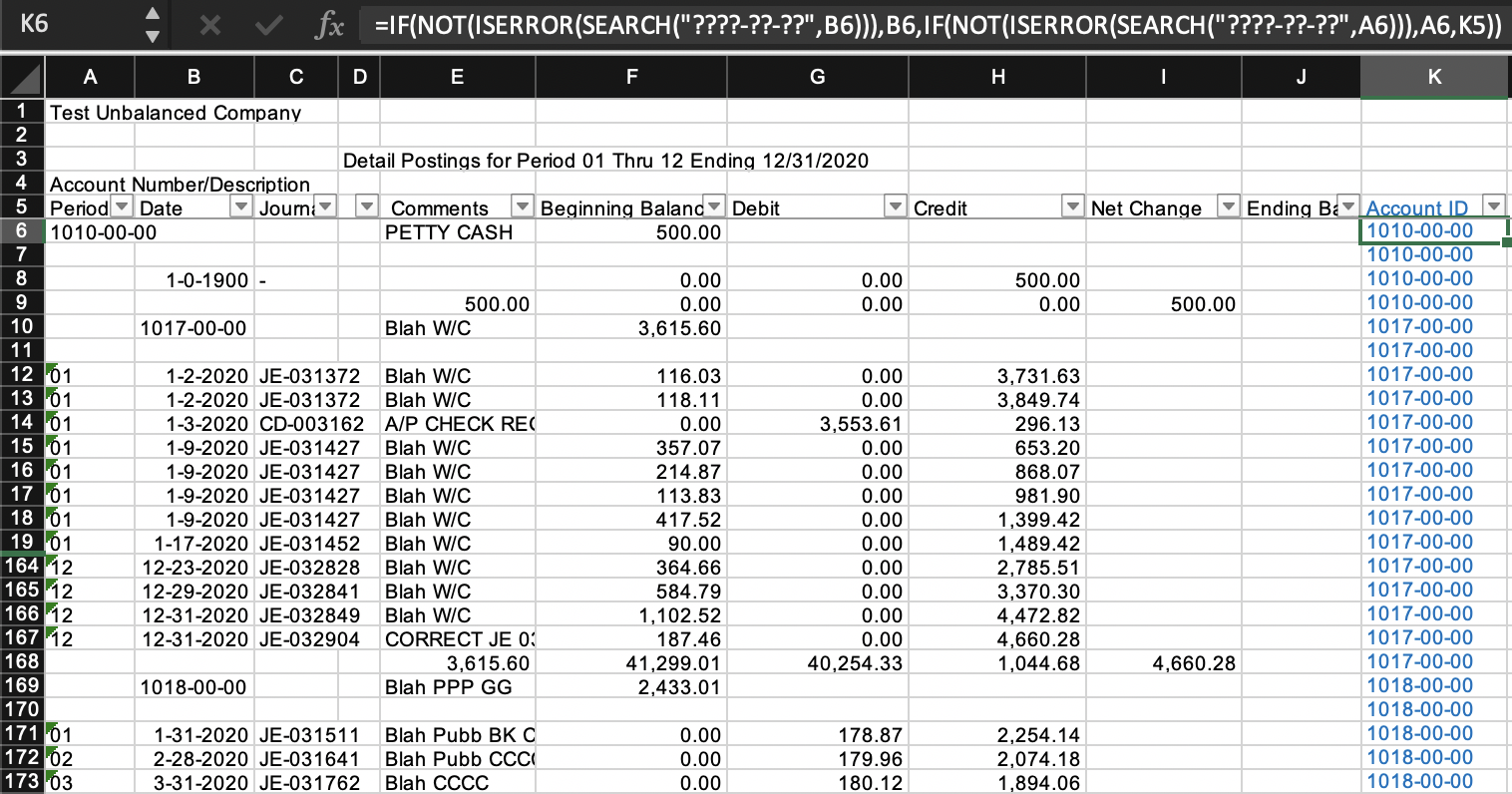### Account ID: Cell K6 and below

`=IF(NOT(ISERROR(SEARCH("????-??-??",B6))),B6,IF(NOT(ISERROR(SEARCH("????-??-??",A6))),A6,K5))`
• `SEARCH("????-??-??",B6),   SEARCH("????-??-??",A6)`
find the position in the string B6 or A6 where a string matching the expression "????-??-??" appears. If it is not found, an error (#VALUE!) is returned
• the expression "????-??-??" uses the wildcard character ? which matches any single character. So "????-??-??" means any four single characters, followed by -, followed by any two single characters, followed by -, followed by any two single characters. The purpose is to test if an account number is found in the cell (B6 or A6)
• The NOT(ISERROR()) wrapped around these SEARCH() expressions just indicate whether cells B6 or A6 contain account numbers (i.e., the "????-??-??" pattern was found). So
`IF(NOT(ISERROR(SEARCH("????-??-??",B6))),B6,`
means to return B6 if B6 is an account numbers, and the
`IF(NOT(ISERROR(SEARCH("????-??-??",A6))),A6,`
that follows means else if A6 is an account number, return A6, and finally, the K5 at the end means otherwise return K5 (the account ID calculated in the row above it, i.e., this is what drags down the account numbers found)
Note: We could also add an "Account Description" column in column L to extract the account description, but note that account description is not needed in the GL if it is present in the chart of accounts; for brevity, we will not discuss in detail adding Account Description, but if we wanted to do so, the logic would be the same as the above for Account ID, but since the account description is always in column E if either column A or column B has an account number, we use the OR() function to test if either B6 or A6 is an account number, and return E6 if yes, L5 (the account description calculated in the row above it, to drag down) for the following formula in cell L6:
`=IF(OR(NOT(ISERROR(SEARCH("????-??-??",B6))),NOT(ISERROR(SEARCH("????-??-??",A6)))),E6,L5)`

### Filter to keep only transaction rows

With filters turned on, check column B (Date) and we see that there are the 2020 and 1900 dates, then the rest are account numbers: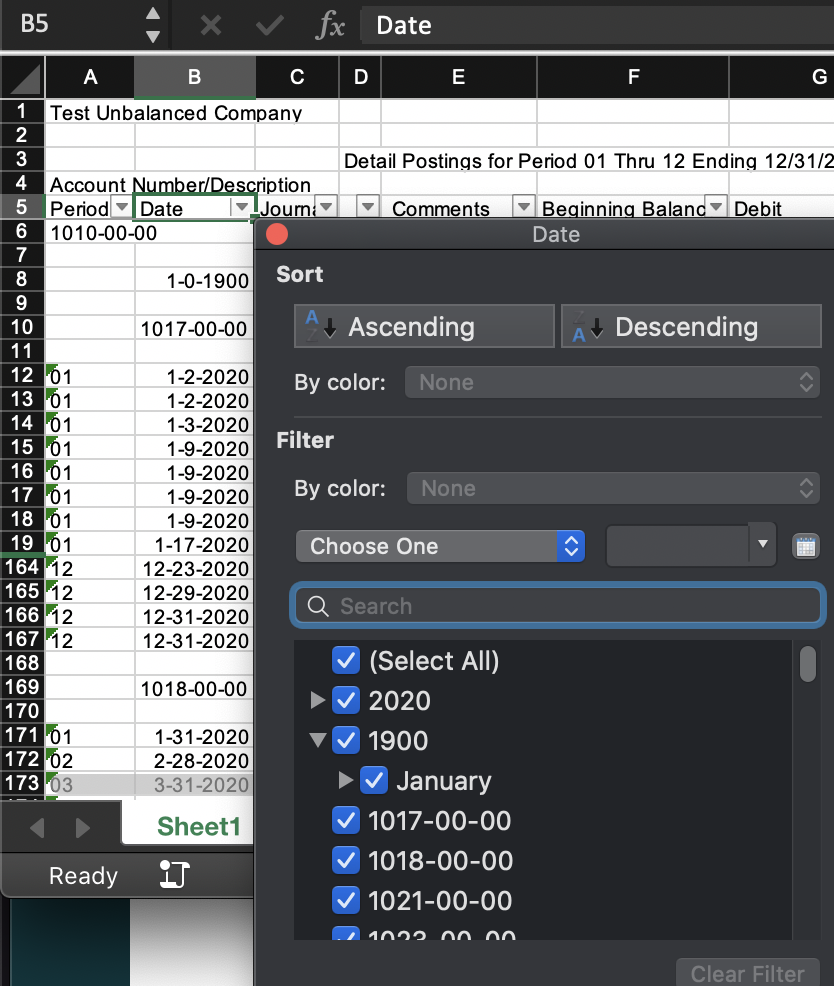It turns out that in this file, the 1900 dates are also garbage data that we do not want to keep, so we will also exclude them in the next step.

### Copy and paste values into a new workbook

Noting that all the 2020 dates are the transaction rows, select only the 2020 dates: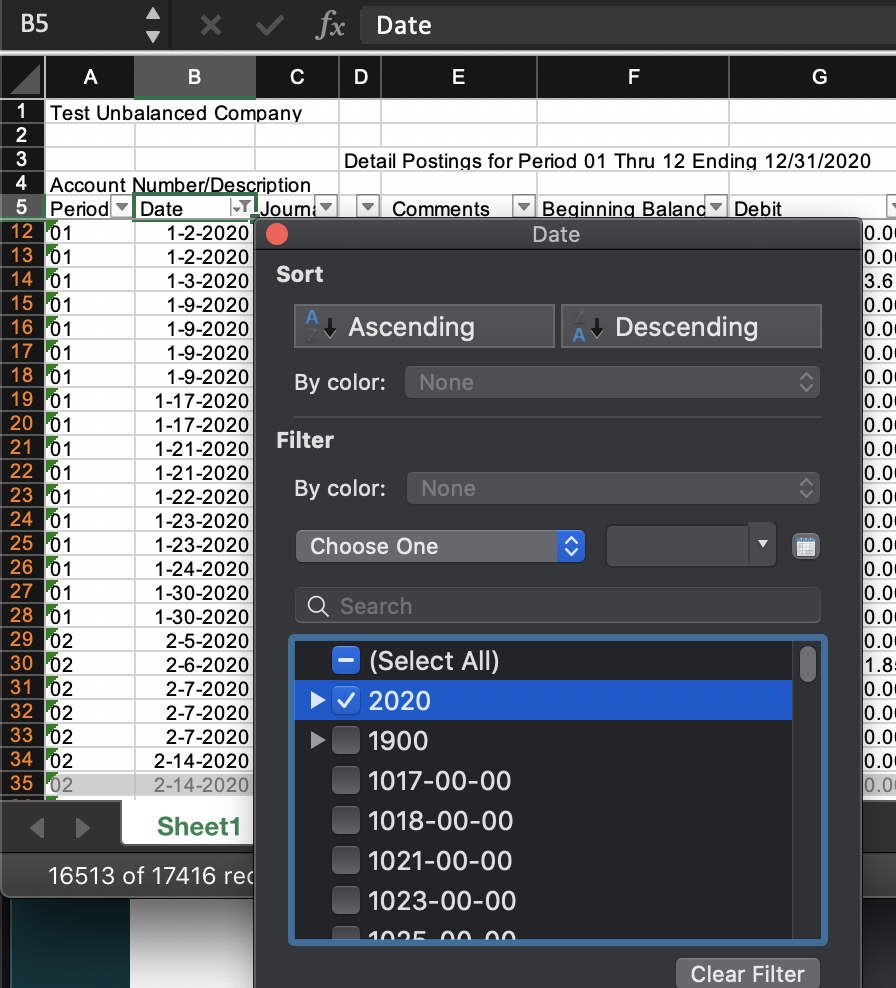Then select the header row and selected transaction rows (i.e., row 5 onward in the filtered view - you can start at cell A5, hit Ctrl-Shift-End to get to the end of the file, and adjust the position as necessary), then copy, then paste special values in a new workbook.

## Troubleshooting the imbalance

So now we have a nicely formatted file that looks like this: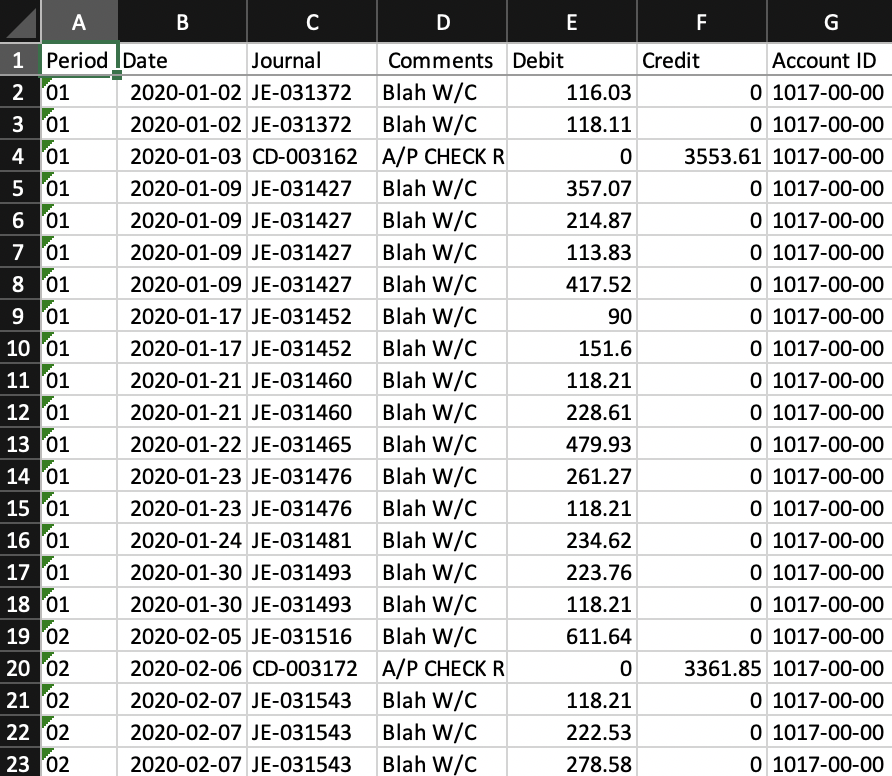But we find that it is out of balance because the debits do not equal the credits!

### Extract account subtotals

Fortunately, we notice that this file has account subtotals (rows that look like row 168 in the first image at the top of this page). And the way we identify these account subtotal rows is that Date (column B) is blank, and Net Change (column I) is a number (i.e., not blank).

So back in the original GL file where we added the formula to column K to extract and drag Account ID down, and with filters still turned on, we filter on Date (column B) is blank and column I is not blank: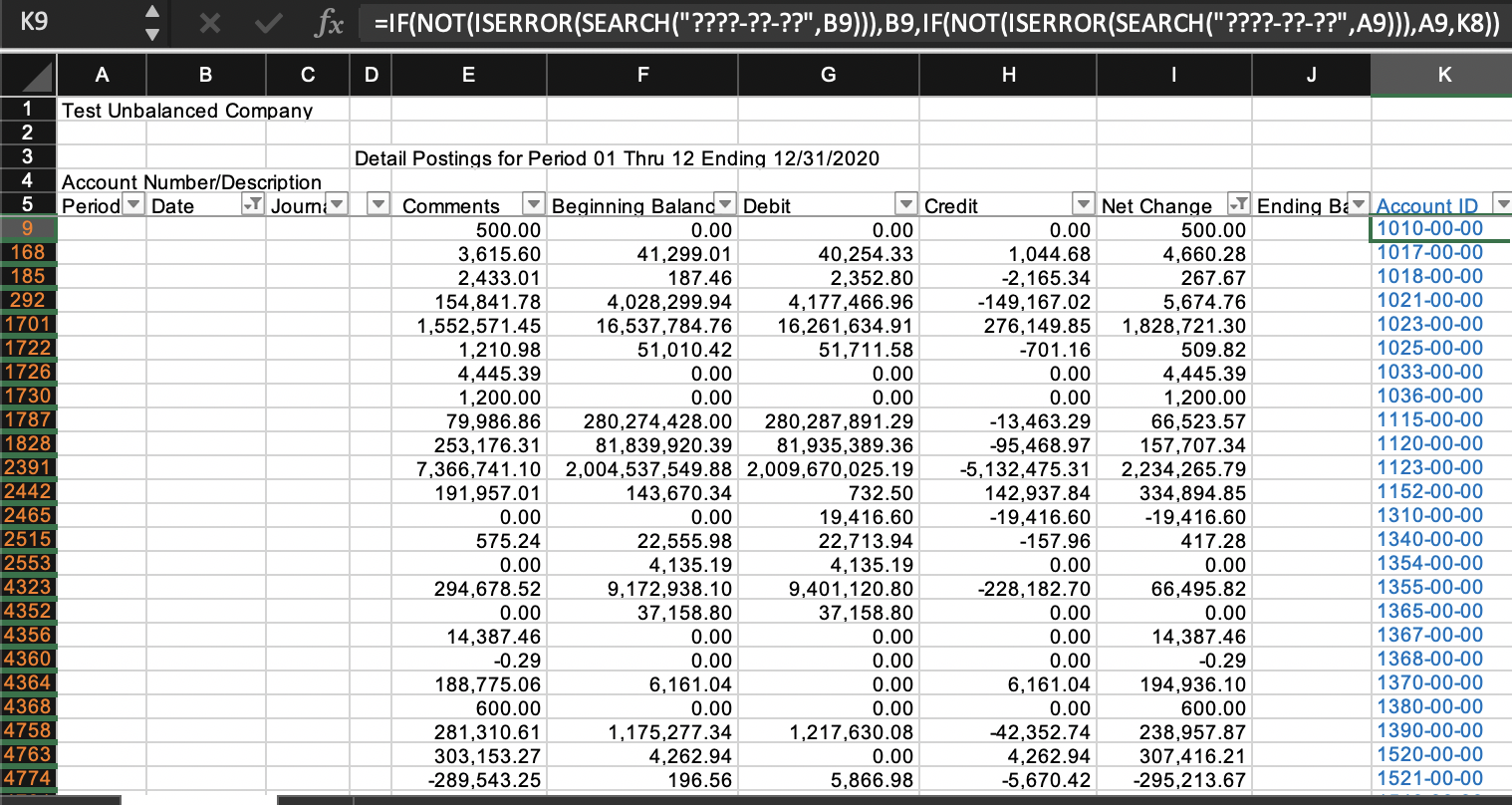Note that the actual columns for these account subtotal rows don't correspond with the column headings - they are in a slightly different format from the transaction rows, one column shifted to the left - Beginning Balance is actually in column E and Ending Balance is actually in column I. But since we are not formatting the subtotals for import into MindBridge, that is okay. We only need to copy and paste values the columns we need (columns E-I and K) into a new worksheet in the workbook with the formatted GL that we will use to troubleshoot the imbalance.

We will name this worksheet subtotals and rename the headers to avoid confusion: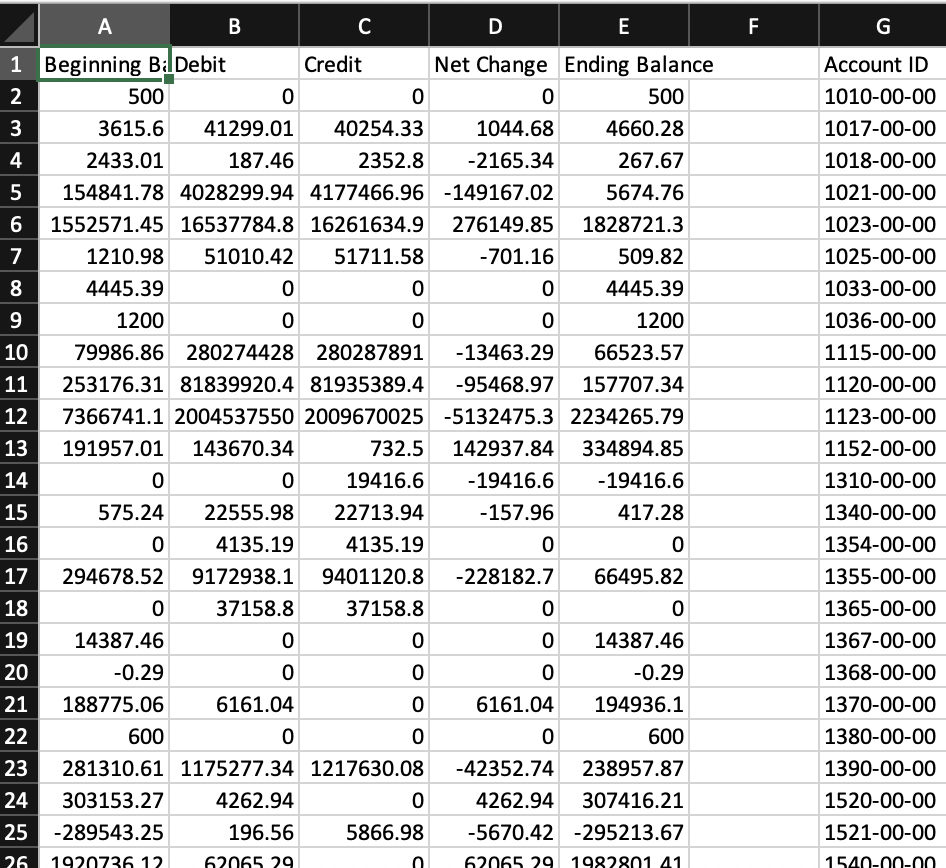### Pivot table on the formatted GL data

Next, we create a pivot table on the formatted GL data in a new worksheet, totaling Debits and Credits by account number:

1. Select all the formatted data (you can start at the top and hit Ctrl-Shift-End to get to the end of the file, and adjust the position as necessary), go to Insert -> Tables -> PivotTableThe Table/Range: will automatically indicate the range you selected for the data (in our case, we have named the worksheet with the formatted GL data "formattedGL" and the data goes from A1 to G16519)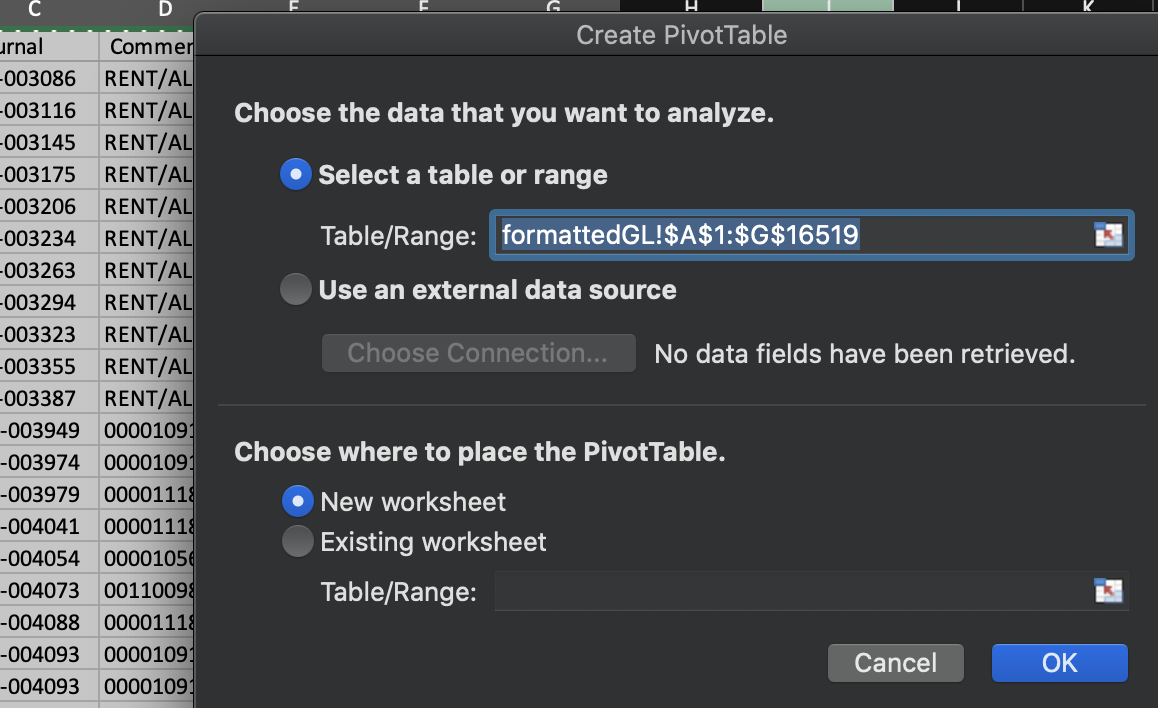2. Keep the "New worksheet" radio button selected (which it should be by default), then click OK.
This will take you to a new worksheet looking like this: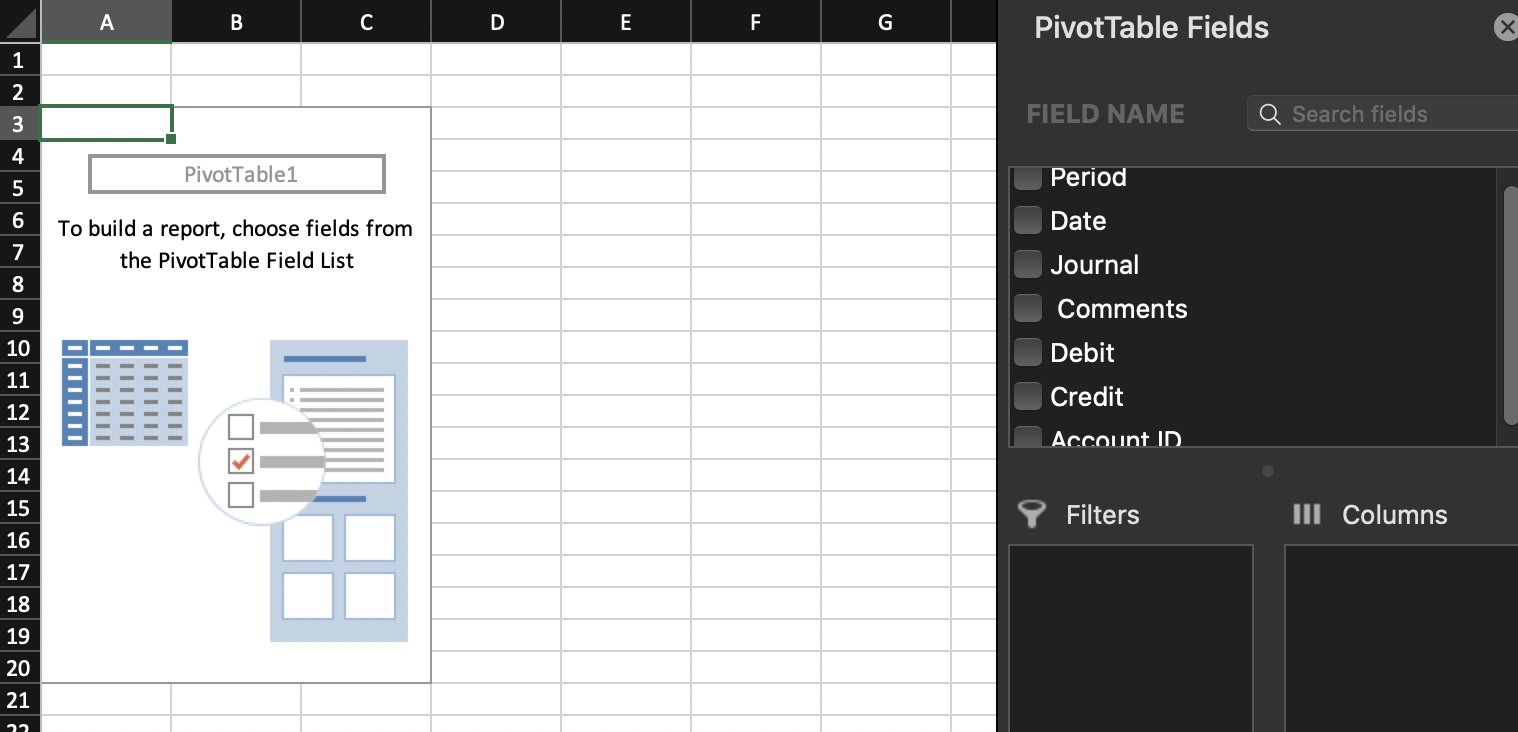We want to total Debit and Credit by Account ID
.

3. Scroll down the FIELD NAME list and click and drag the "Account ID" to the Rows section:4. Then drag Debit and Credit to the Values section:5. Now your pivot table should look like this: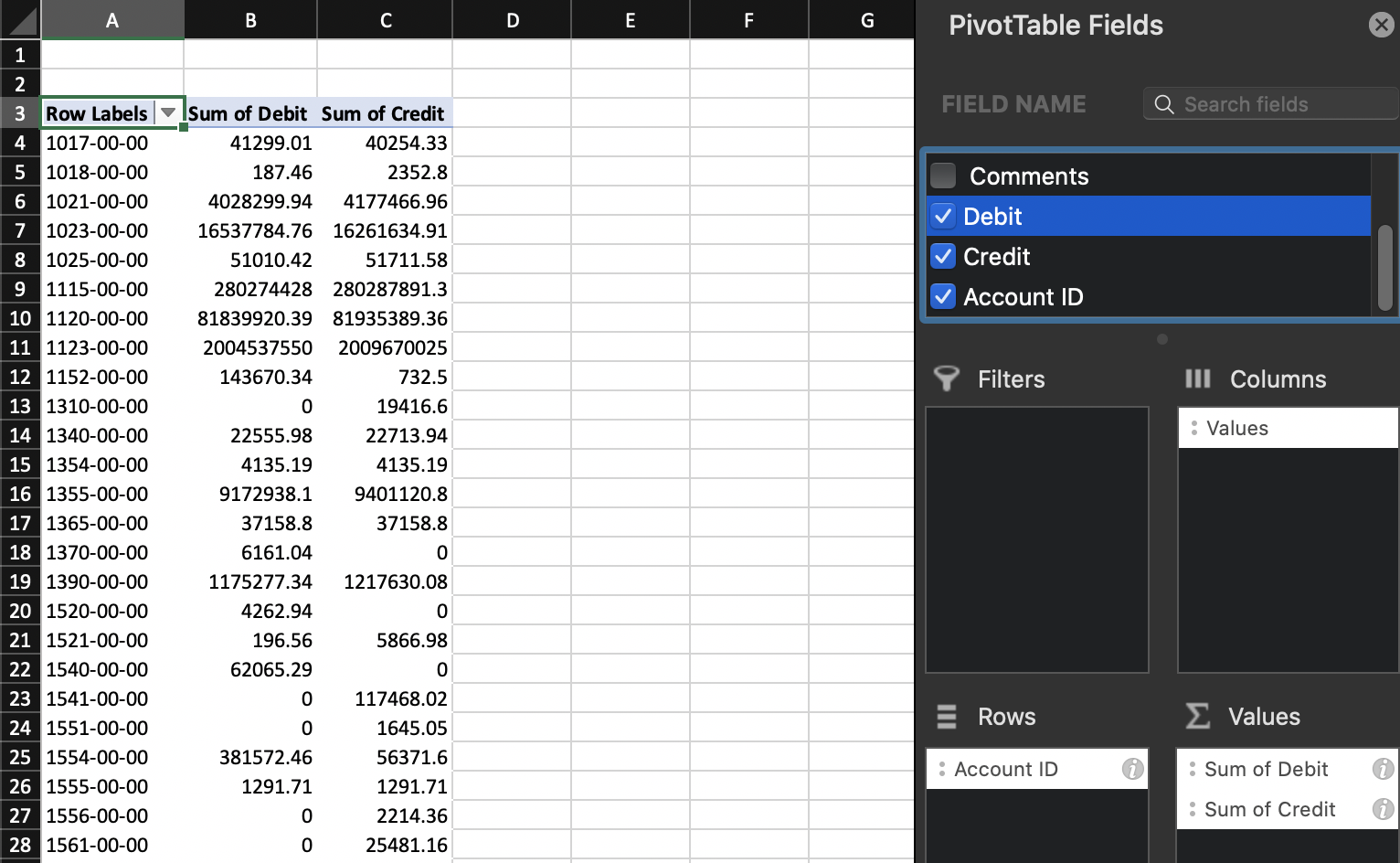### Lookup and compare with account subtotals

Now we want to compare these account totals with the ones that we had extracted from the original GL and saved in the subtotals worksheet: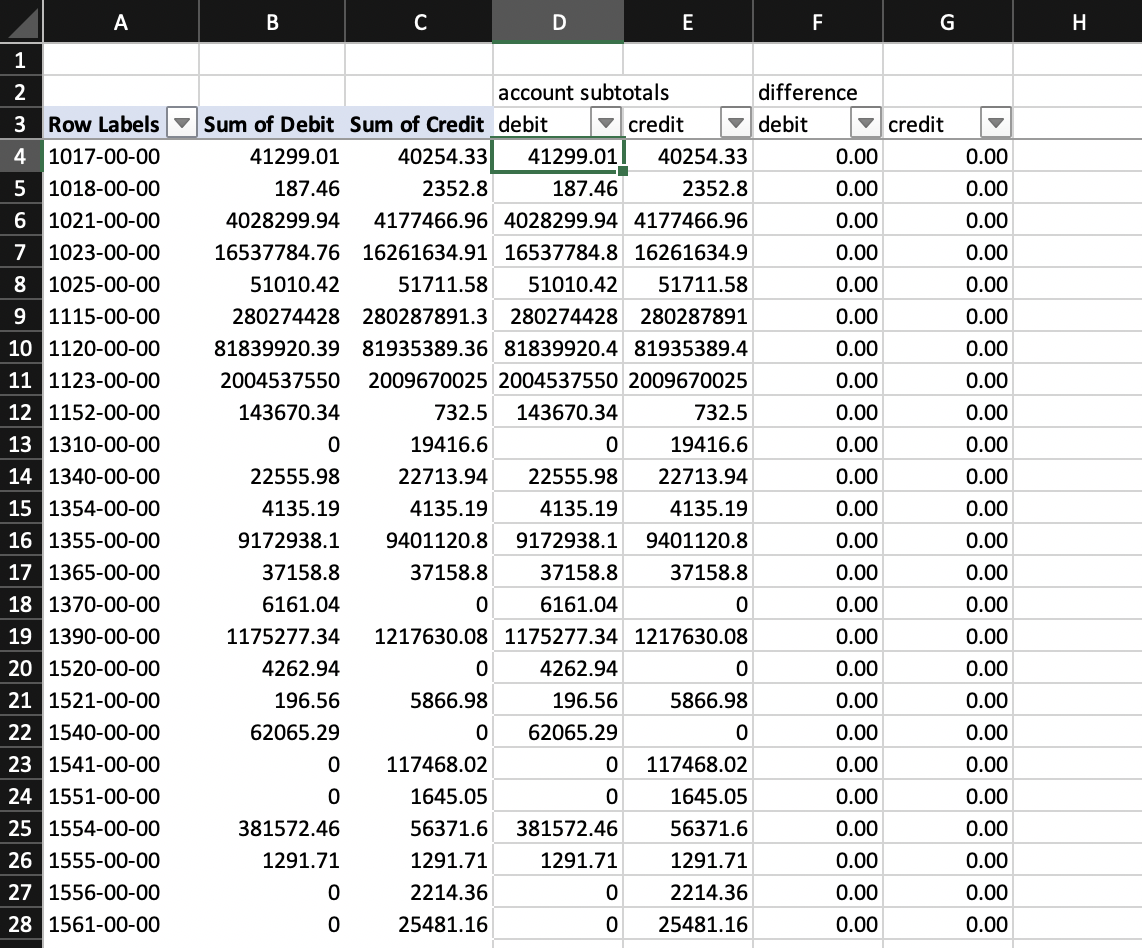#### Account subtotals: debit column formula

`=SUMIF(subtotals!\$G:\$G,\$A4,subtotals!B:B)`

Recall that in the subtotals worksheet, column G has the Account ID and columns B and C the Debit and Credit amounts: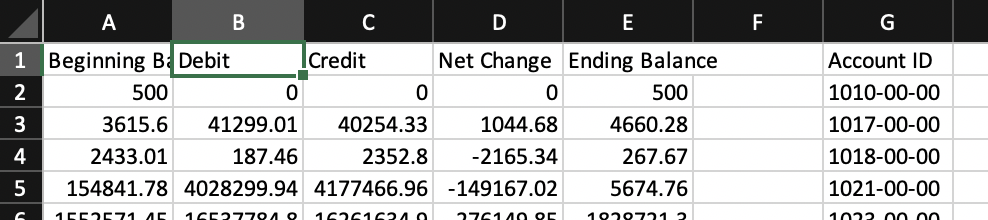Column A in the pivot table worksheet has the Account IDs. So this SUMIF formula gets the debit and credit totals from the subtotals worksheet for each account ID in the pivot table.

#### account subtotals: credit column formula (just copy and paste the debit column formula)

`=SUMIF(subtotals!\$G:\$G,\$A4,subtotals!C:C)`

Finally, add the formulas in columns F and G:

```=B4-D4
=C4-E4```

Select columns D-G and add filters:We see that there are some differences in the debit column: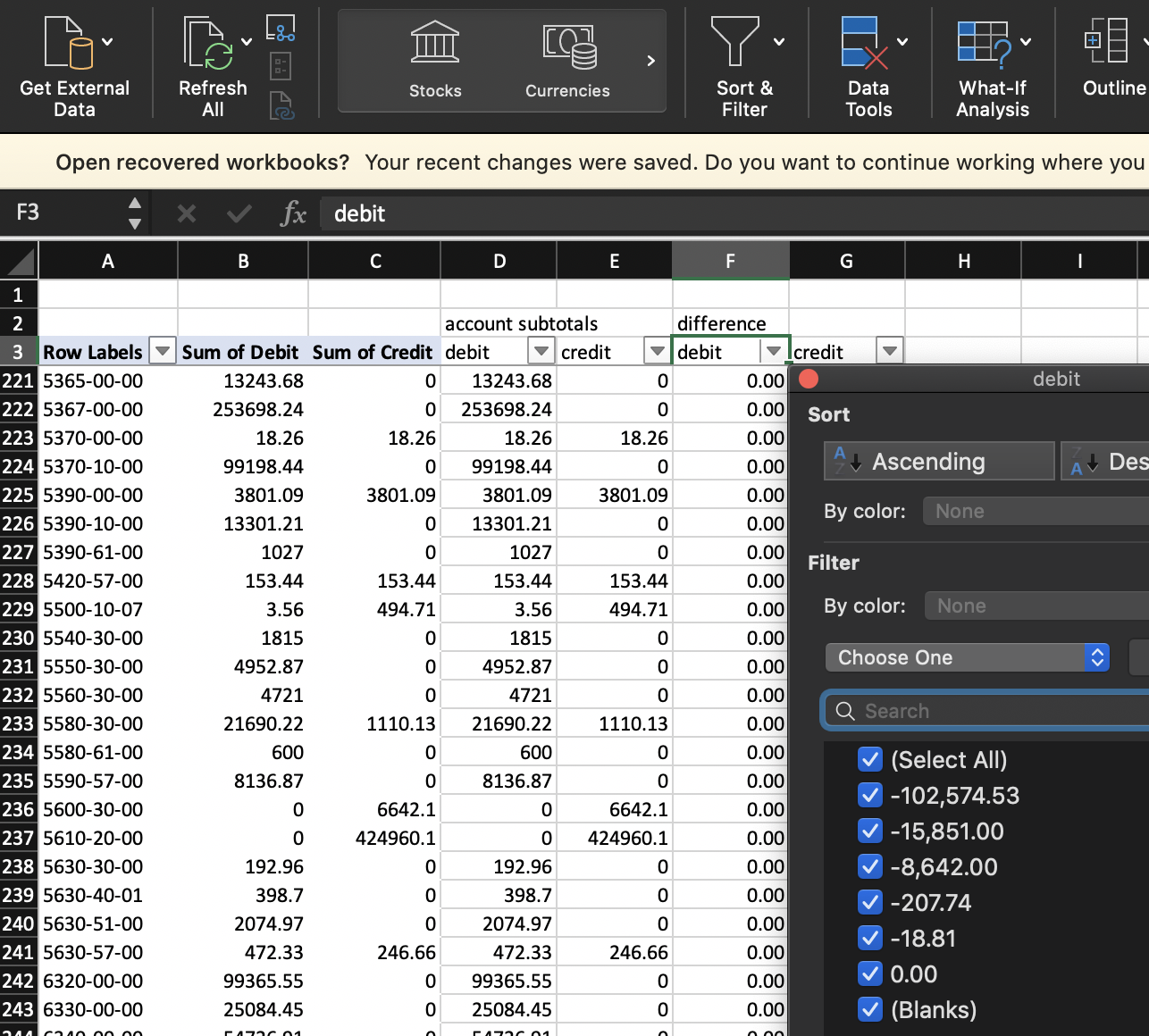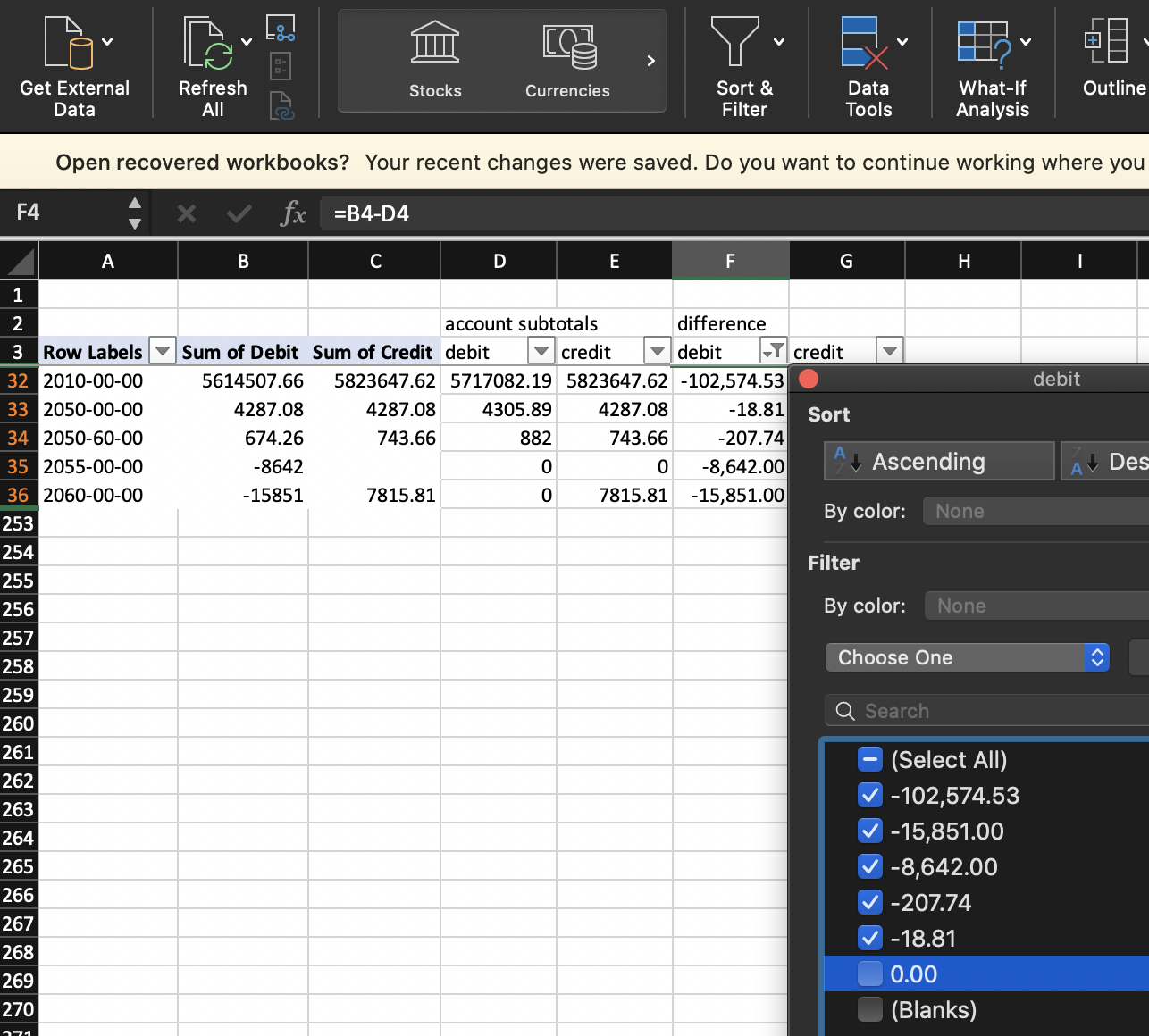Now we have identified account numbers to investigate for differences.

### Comparing subtotals with formatted GL starting from the subtotals

Note that if there are account numbers in the original GLs subtotals list that are not in the formatted GL, those won't appear here but could account for differences. To detect those, you can run add to the subtotals worksheet SUMIF() formulas referencing the formatted GL to pull the account totals from the formatted GL: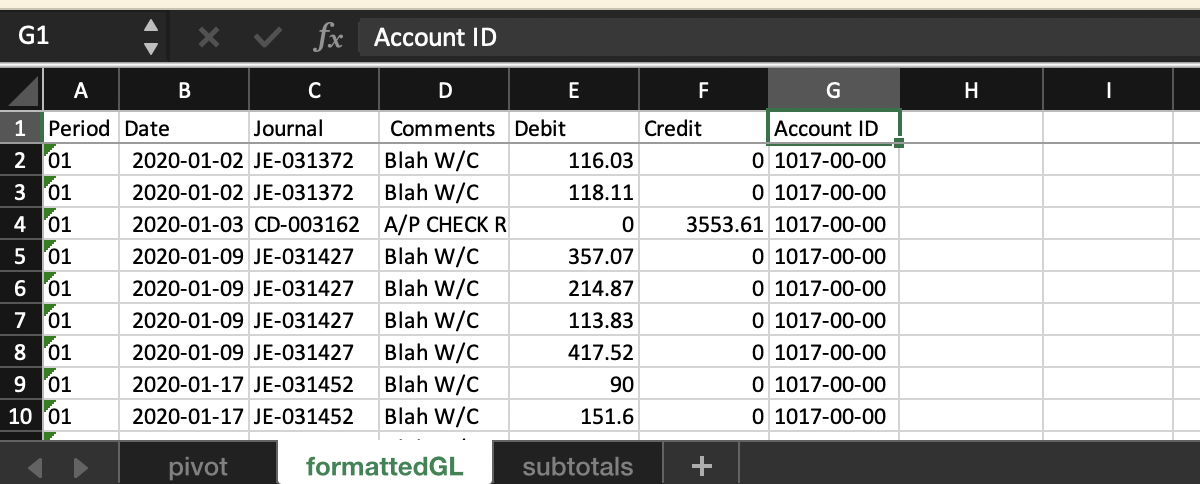Anything else on your mind? Chat with us or submit a request for further assistance.